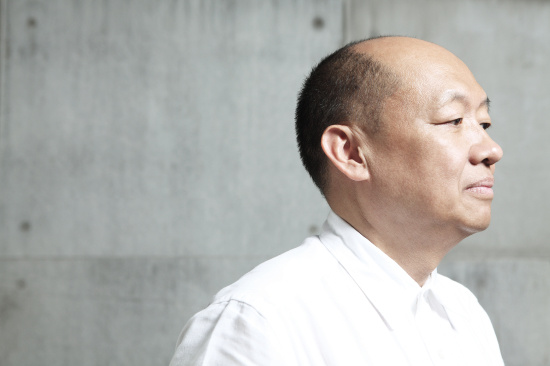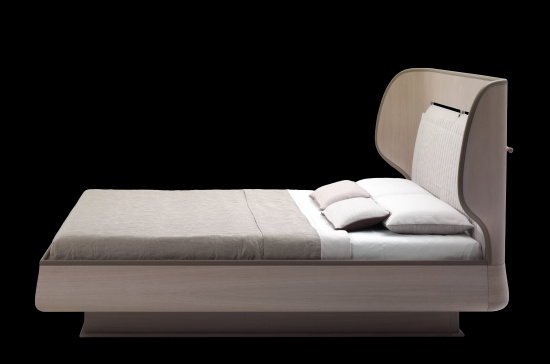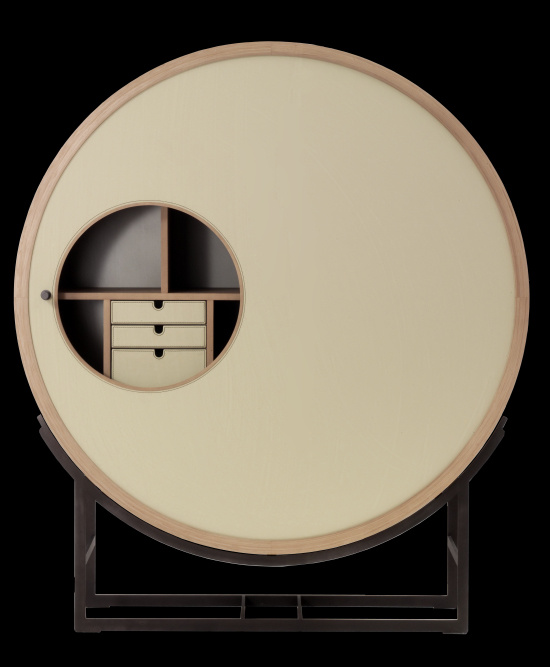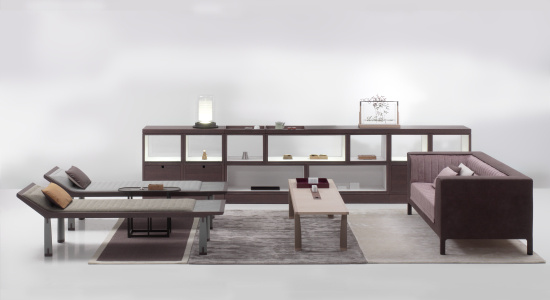|

# 多款原创设计 高调亮相米兰国际家具展(组图)

ACF CHI WING LO是中国ACF设计产业集团与国际著名建筑、空间、家居产品设计大师卢志荣先生共同创立的设计师联合品牌。全部产品均由卢志荣先生亲自设计，并在意大利顶级制造工厂中结合现代机械工艺和意大利传统手工技艺精心打造而成，旨在为消费者带来最纯粹的国际设计、意大利工艺、品质的完整体验，成就用家具打造卓越生活的梦想。卢志荣近影X

ACF CHI WING LO设计师卢志荣先生活跃于意大利的设计领域近二十年，是唯一一位被久负盛名的意大利设计传统圈认可的华裔建筑师、设计师及雕塑艺术家。曾任意大利百年家俬品牌 Giorgetti 首席设计师近20年，是这个意大利国宝级品牌合作的第一位华人设计师。他的创造领域综合了艺术、建筑、室内、家具设计，他提出的“永恒的设计”的理念得到国际广泛的关注、以至到今天许多早年的作品依然被追捧。卢志荣先生在设计上独特的追求，在工艺上对细节的精准把控，以及在材料选用上的创新，为家具创造了新的标准以及新的期待。他对家具设计的衷爱和热情就是ACF CHI WING LO品牌最可信赖的署名。X

ACF CHI WING LO是中国ACF设计产业集团与国际著名建筑、空间、家居产品设计大师卢志荣先生共同创立的设计师联合品牌。全部产品均由卢志荣先生亲自设计，并在意大利顶级制造工厂中结合现代机械工艺和意大利传统手工技艺精心打造而成，旨在为消费者带来最纯粹的国际设计、意大利工艺、品质的完整体验，成就用家具打造卓越生活的梦想。X

ACF CHI WING LO设计师卢志荣先生活跃于意大利的设计领域近二十年，是唯一一位被久负盛名的意大利设计传统圈认可的华裔建筑师、设计师及雕塑艺术家。曾任意大利百年家俬品牌 Giorgetti 首席设计师近20年，是这个意大利国宝级品牌合作的第一位华人设计师。他的创造领域综合了艺术、建筑、室内、家具设计，他提出的“永恒的设计”的理念得到国际广泛的关注、以至到今天许多早年的作品依然被追捧。卢志荣先生在设计上独特的追求，在工艺上对细节的精准把控，以及在材料选用上的创新，为家具创造了新的标准以及新的期待。他对家具设计的衷爱和热情就是ACF CHI WING LO品牌最可信赖的署名。X

ACF CHI WING LO是中国ACF设计产业集团与国际著名建筑、空间、家居产品设计大师卢志荣先生共同创立的设计师联合品牌。全部产品均由卢志荣先生亲自设计，并在意大利顶级制造工厂中结合现代机械工艺和意大利传统手工技艺精心打造而成，旨在为消费者带来最纯粹的国际设计、意大利工艺、品质的完整体验，成就用家具打造卓越生活的梦想。X

ACF CHI WING LO设计师卢志荣先生活跃于意大利的设计领域近二十年，是唯一一位被久负盛名的意大利设计传统圈认可的华裔建筑师、设计师及雕塑艺术家。曾任意大利百年家俬品牌 Giorgetti 首席设计师近20年，是这个意大利国宝级品牌合作的第一位华人设计师。他的创造领域综合了艺术、建筑、室内、家具设计，他提出的“永恒的设计”的理念得到国际广泛的关注、以至到今天许多早年的作品依然被追捧。卢志荣先生在设计上独特的追求，在工艺上对细节的精准把控，以及在材料选用上的创新，为家具创造了新的标准以及新的期待。他对家具设计的衷爱和热情就是ACF CHI WING LO品牌最可信赖的署名。X

ACF CHI WING LO是中国ACF设计产业集团与国际著名建筑、空间、家居产品设计大师卢志荣先生共同创立的设计师联合品牌。全部产品均由卢志荣先生亲自设计，并在意大利顶级制造工厂中结合现代机械工艺和意大利传统手工技艺精心打造而成，旨在为消费者带来最纯粹的国际设计、意大利工艺、品质的完整体验，成就用家具打造卓越生活的梦想。X

ACF CHI WING LO设计师卢志荣先生活跃于意大利的设计领域近二十年，是唯一一位被久负盛名的意大利设计传统圈认可的华裔建筑师、设计师及雕塑艺术家。曾任意大利百年家俬品牌 Giorgetti 首席设计师近20年，是这个意大利国宝级品牌合作的第一位华人设计师。他的创造领域综合了艺术、建筑、室内、家具设计，他提出的“永恒的设计”的理念得到国际广泛的关注、以至到今天许多早年的作品依然被追捧。卢志荣先生在设计上独特的追求，在工艺上对细节的精准把控，以及在材料选用上的创新，为家具创造了新的标准以及新的期待。他对家具设计的衷爱和热情就是ACF CHI WING LO品牌最可信赖的署名。`声明：本文由入驻焦点开放平台的作者撰写，除焦点官方账号外，观点仅代表作者本人，不代表焦点立场错误信息举报电话： 400-099-0099，邮箱：jubao@vip.sohu.com，或点此进行意见反馈，或点此进行举报投诉。`A B C D E F G H J K L M N P Q R S T W X Y Z
A - B - C - D - E
• A
• 鞍山
• 安庆
• 安阳
• 安顺
• 安康
• 澳门
• B
• 北京
• 保定
• 包头
• 巴彦淖尔
• 本溪
• 蚌埠
• 亳州
• 滨州
• 北海
• 百色
• 巴中
• 毕节
• 保山
• 宝鸡
• 白银
• 巴州
• C
• 承德
• 沧州
• 长治
• 赤峰
• 朝阳
• 长春
• 常州
• 滁州
• 池州
• 长沙
• 常德
• 郴州
• 潮州
• 崇左
• 重庆
• 成都
• 楚雄
• 昌都
• 慈溪
• 常熟
• D
• 大同
• 大连
• 丹东
• 大庆
• 东营
• 德州
• 东莞
• 德阳
• 达州
• 大理
• 德宏
• 定西
• 儋州
• 东平
• E
• 鄂尔多斯
• 鄂州
• 恩施
F - G - H - I - J
• F
• 抚顺
• 阜新
• 阜阳
• 福州
• 抚州
• 佛山
• 防城港
• G
• 赣州
• 广州
• 桂林
• 贵港
• 广元
• 广安
• 贵阳
• 固原
• H
• 邯郸
• 衡水
• 呼和浩特
• 呼伦贝尔
• 葫芦岛
• 哈尔滨
• 黑河
• 淮安
• 杭州
• 湖州
• 合肥
• 淮南
• 淮北
• 黄山
• 菏泽
• 鹤壁
• 黄石
• 黄冈
• 衡阳
• 怀化
• 惠州
• 河源
• 贺州
• 河池
• 海口
• 红河
• 汉中
• 海东
• 怀来
• I
• J
• 晋中
• 锦州
• 吉林
• 鸡西
• 佳木斯
• 嘉兴
• 金华
• 景德镇
• 九江
• 吉安
• 济南
• 济宁
• 焦作
• 荆门
• 荆州
• 江门
• 揭阳
• 金昌
• 酒泉
• 嘉峪关
K - L - M - N - P
• K
• 开封
• 昆明
• 昆山
• L
• 廊坊
• 临汾
• 辽阳
• 连云港
• 丽水
• 六安
• 龙岩
• 莱芜
• 临沂
• 聊城
• 洛阳
• 漯河
• 娄底
• 柳州
• 来宾
• 泸州
• 乐山
• 六盘水
• 丽江
• 临沧
• 拉萨
• 林芝
• 兰州
• 陇南
• M
• 牡丹江
• 马鞍山
• 茂名
• 梅州
• 绵阳
• 眉山
• N
• 南京
• 南通
• 宁波
• 南平
• 宁德
• 南昌
• 南阳
• 南宁
• 内江
• 南充
• P
• 盘锦
• 莆田
• 平顶山
• 濮阳
• 攀枝花
• 普洱
• 平凉
Q - R - S - T - W
• Q
• 秦皇岛
• 齐齐哈尔
• 衢州
• 泉州
• 青岛
• 清远
• 钦州
• 黔南
• 曲靖
• 庆阳
• R
• 日照
• 日喀则
• S
• 石家庄
• 沈阳
• 双鸭山
• 绥化
• 上海
• 苏州
• 宿迁
• 绍兴
• 宿州
• 三明
• 上饶
• 三门峡
• 商丘
• 十堰
• 随州
• 邵阳
• 韶关
• 深圳
• 汕头
• 汕尾
• 三亚
• 三沙
• 遂宁
• 山南
• 商洛
• 石嘴山
• T
• 天津
• 唐山
• 太原
• 通辽
• 铁岭
• 泰州
• 台州
• 铜陵
• 泰安
• 铜仁
• 铜川
• 天水
• 天门
• W
• 乌海
• 乌兰察布
• 无锡
• 温州
• 芜湖
• 潍坊
• 威海
• 武汉
• 梧州
• 渭南
• 武威
• 吴忠
• 乌鲁木齐
X - Y - Z
• X
• 邢台
• 徐州
• 宣城
• 厦门
• 新乡
• 许昌
• 信阳
• 襄阳
• 孝感
• 咸宁
• 湘潭
• 湘西
• 西双版纳
• 西安
• 咸阳
• 西宁
• 仙桃
• 西昌
• Y
• 运城
• 营口
• 盐城
• 扬州
• 鹰潭
• 宜春
• 烟台
• 宜昌
• 岳阳
• 益阳
• 永州
• 阳江
• 云浮
• 玉林
• 宜宾
• 雅安
• 玉溪
• 延安
• 榆林
• 银川
• Z
• 张家口
• 镇江
• 舟山
• 漳州
• 淄博
• 枣庄
• 郑州
• 周口
• 驻马店
• 株洲
• 张家界
• 珠海
• 湛江
• 肇庆
• 中山
• 自贡
• 资阳
• 遵义
• 昭通
• 张掖
• 中卫

1室1厅1厨1卫1阳台

1
2
3
4
5

0
1
2

1

1

0
1
2
3报名成功，资料已提交审核A B C D E F G H J K L M N P Q R S T W X Y Z
A - B - C - D - E
• A
• 鞍山
• 安庆
• 安阳
• 安顺
• 安康
• 澳门
• B
• 北京
• 保定
• 包头
• 巴彦淖尔
• 本溪
• 蚌埠
• 亳州
• 滨州
• 北海
• 百色
• 巴中
• 毕节
• 保山
• 宝鸡
• 白银
• 巴州
• C
• 承德
• 沧州
• 长治
• 赤峰
• 朝阳
• 长春
• 常州
• 滁州
• 池州
• 长沙
• 常德
• 郴州
• 潮州
• 崇左
• 重庆
• 成都
• 楚雄
• 昌都
• 慈溪
• 常熟
• D
• 大同
• 大连
• 丹东
• 大庆
• 东营
• 德州
• 东莞
• 德阳
• 达州
• 大理
• 德宏
• 定西
• 儋州
• 东平
• E
• 鄂尔多斯
• 鄂州
• 恩施
F - G - H - I - J
• F
• 抚顺
• 阜新
• 阜阳
• 福州
• 抚州
• 佛山
• 防城港
• G
• 赣州
• 广州
• 桂林
• 贵港
• 广元
• 广安
• 贵阳
• 固原
• H
• 邯郸
• 衡水
• 呼和浩特
• 呼伦贝尔
• 葫芦岛
• 哈尔滨
• 黑河
• 淮安
• 杭州
• 湖州
• 合肥
• 淮南
• 淮北
• 黄山
• 菏泽
• 鹤壁
• 黄石
• 黄冈
• 衡阳
• 怀化
• 惠州
• 河源
• 贺州
• 河池
• 海口
• 红河
• 汉中
• 海东
• 怀来
• I
• J
• 晋中
• 锦州
• 吉林
• 鸡西
• 佳木斯
• 嘉兴
• 金华
• 景德镇
• 九江
• 吉安
• 济南
• 济宁
• 焦作
• 荆门
• 荆州
• 江门
• 揭阳
• 金昌
• 酒泉
• 嘉峪关
K - L - M - N - P
• K
• 开封
• 昆明
• 昆山
• L
• 廊坊
• 临汾
• 辽阳
• 连云港
• 丽水
• 六安
• 龙岩
• 莱芜
• 临沂
• 聊城
• 洛阳
• 漯河
• 娄底
• 柳州
• 来宾
• 泸州
• 乐山
• 六盘水
• 丽江
• 临沧
• 拉萨
• 林芝
• 兰州
• 陇南
• M
• 牡丹江
• 马鞍山
• 茂名
• 梅州
• 绵阳
• 眉山
• N
• 南京
• 南通
• 宁波
• 南平
• 宁德
• 南昌
• 南阳
• 南宁
• 内江
• 南充
• P
• 盘锦
• 莆田
• 平顶山
• 濮阳
• 攀枝花
• 普洱
• 平凉
Q - R - S - T - W
• Q
• 秦皇岛
• 齐齐哈尔
• 衢州
• 泉州
• 青岛
• 清远
• 钦州
• 黔南
• 曲靖
• 庆阳
• R
• 日照
• 日喀则
• S
• 石家庄
• 沈阳
• 双鸭山
• 绥化
• 上海
• 苏州
• 宿迁
• 绍兴
• 宿州
• 三明
• 上饶
• 三门峡
• 商丘
• 十堰
• 随州
• 邵阳
• 韶关
• 深圳
• 汕头
• 汕尾
• 三亚
• 三沙
• 遂宁
• 山南
• 商洛
• 石嘴山
• T
• 天津
• 唐山
• 太原
• 通辽
• 铁岭
• 泰州
• 台州
• 铜陵
• 泰安
• 铜仁
• 铜川
• 天水
• 天门
• W
• 乌海
• 乌兰察布
• 无锡
• 温州
• 芜湖
• 潍坊
• 威海
• 武汉
• 梧州
• 渭南
• 武威
• 吴忠
• 乌鲁木齐
X - Y - Z
• X
• 邢台
• 徐州
• 宣城
• 厦门
• 新乡
• 许昌
• 信阳
• 襄阳
• 孝感
• 咸宁
• 湘潭
• 湘西
• 西双版纳
• 西安
• 咸阳
• 西宁
• 仙桃
• 西昌
• Y
• 运城
• 营口
• 盐城
• 扬州
• 鹰潭
• 宜春
• 烟台
• 宜昌
• 岳阳
• 益阳
• 永州
• 阳江
• 云浮
• 玉林
• 宜宾
• 雅安
• 玉溪
• 延安
• 榆林
• 银川
• Z
• 张家口
• 镇江
• 舟山
• 漳州
• 淄博
• 枣庄
• 郑州
• 周口
• 驻马店
• 株洲
• 张家界
• 珠海
• 湛江
• 肇庆
• 中山
• 自贡
• 资阳
• 遵义
• 昭通
• 张掖
• 中卫• 手机• 分享
• 设计
免费设计
• 计算器
装修计算器
• 入驻
合作入驻
• 联系
联系我们
• 置顶
返回顶部